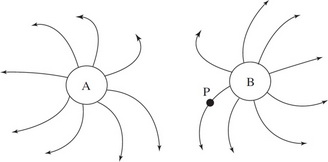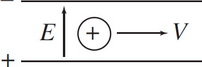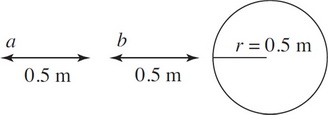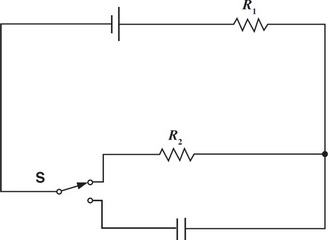# AP Physics 2 Practice Test 9

### Test Information10 questions18 minutes

1.The diagram above shows the electric field lines around a region of space due to two charges A and B. Which of the following statements is true?

2.A particle travels through a uniform electric field E = 5 N/C as shown. What is the size and direction of the magnetic field needed to have a 0.2 C charge with mass 0.005 kg pass through undeflected at a velocity of 4 m/s?

3.A hollow sphere carries a charge of 3 C. How does the magnitude of the field at point a compare to point b ?

4. A solid plastic cube with uniform density (side length = 0.5 m) of mass 100 kg is placed in a vat of fluid whose density is 1200 kg/m3. What fraction of the cube's volume floats above the surface of the fluid?

5. A point charge, Q = +1 mC, is fixed at the origin. How much work is required to move a charge, Q = +8 µC, from the point (0, 4 meters) to the point (3 meters, 0)?

6. The following three steps process refers to a simple RC circuit with a battery and an initially open switch.

Step 1: The switch is closed, allowing the capacitor to charge.

Step 2: After the capacitor has charged, a slab of dielectric material is inserted between the plates of the capacitor and time passes.

Step 3: The switch is opened, and the dielectric is removed.

Which of the following describes the change in potential energy stored in the capacitor during each step?

7. An electron (mass = m, charge = -E) is rotated with speed V upwards, in the plane of the page, into a region containing a uniform magnetic field B, that is directed into the plane of the page. Describe the electron's subsequent circular motion.

8.When switch is in the position shown in the figure, what is the current through the circuit? The voltage of the battery is 10 V, R1 = 2 Ω and R2 = 3 Ω.

9.When the switch is moved to the opposite position, which of the following statements descirbed the current through the circuit. Consider the capacitor as starting with no charge.

10. Optometrists use a linear scale of lens power, measured in diopters, to prescribe corrective lenses. Sufferers of myopia, or nearsightedness, have difficulty resolving distant objects because the lenses in their eyes are too strong. If a myopic person has a prescription of -5 diopters, what image do her corrective lenses create for distant objects?